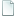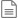Topic: Ambit.vbs - Show notes found in selection (Read 8039 times)

## Ambit.vbs - Show notes found in selection##### 2009-04-10 03:09 pm
This program shows the notes used. Ambit is the anglicized form of Ambitus. Usually this is used to find the range for a vocalist but, in my extended form, more useful data is provided.

After MIDI import, transposition or shifting of notes, one is usually must go through the entire staff to check for stange enharmonic note spellings. After fixing them, another search is usually needed to verify. This tool can expedite this.

It produces a short series of muted grace note clusters representing all the note/acccidental positions occurring in the selection. This varies if accidentals are forced. Both the forced and unforced results are useful.
Quote from: Ambit.vbs
Option Explicit ' Ambit.vbs, ver 1.1
' NWC2 UserTool to show all notes used in selection
' &Command: WScript Scripts\Ambit.vbs
' Reference: https://forum.noteworthycomposer.com/?topic=6786.0
' History:
' 2009-12-28: 2.1 Beta 5 aware (1.1)
' 2009-04-10: Initial release (1.0)
Const Template = "|Chord|Dur:Whole,Grace|Pos:?|Opts:Muted"
Dim d: Set d = CreateObject("Scripting.Dictionary")
Dim a1, a2, e, i, s
For Each e In Array("", "n", "b", "#", "x", "v")
Set d(e) = CreateObject("Scripting.Dictionary")
Next
Dim aIn: aIn = Split(WScript.StdIn.ReadAll, vbCrLf)
For i = 1 To UBound(aIn) - 2
a1 = Split(aIn(i), "|")
Select Case a1(1)
Case "Note", "Chord", "RestChord"
For Each e In Filter(a1, "Pos")
For Each s In Split(Split(e, ":")(1), ",")
a2 = aPos5(s)
d(a2(0))(a2(0) & a2(1)) = ""
Next
Next
End Select
Next
For Each e In d
s = Join(d(e).Keys, ",")
If Len(s) Then aIn(0) = aIn(0) & vbCrLf & Replace(Template, "?", s)
Next
If InStr(aIn(0), vbLf) Then aIn(0) = aIn(0) & vbCrLf & "|Bar|XBarCnt:Y"
WScript.Stdout.Write Join(aIn, vbCrLf)

Function aPos5(ByVal s) ' NotePos to array
Dim r(4), e ' retval, element
If InStr("nb#xv", Left(s, 1)) Then r(0) = Left(s, 1) ' accidental
If InStr(s, "^") Then  r(3) = "^" ' tie
If InStr(s, "!") Then r(4) = Mid(s, InStr(s, "!"), 2) ' color
For Each e In r: s = Replace(s, e, "", 1, 1): Next
If Not IsNumeric(s) Then r(2) = Right(s, 1) ' notehead
r(1) = Replace(s, r(2), "")
aPos5 = r ' acc, pos, head, tie, color
End Function

Rose.png shows the result for the Vocal staff of the sample file: Rose.nwc

Edit: 2009-12-28: made 2.1 Beta 5 aware (1.1), older version below.

`Option Explicit ' Ambit.vbs, ver 1.0' NWC2 UserTool to show all notes used in selection' &Command: WScript Scripts\Ambit.vbsConst Template = "|Chord|Dur:Whole,Grace|Pos:?|Opts:Muted"Dim d: Set d = CreateObject("Scripting.Dictionary")Dim a1, a2, e, i, sFor Each e In Array("", "n", "b", "#", "x", "v")  Set d(e) = CreateObject("Scripting.Dictionary")NextDim aIn: aIn = Split(WScript.StdIn.ReadAll, vbCrLf)For i = 1 To UBound(aIn) - 2  a1 = Split(aIn(i), "|")  Select Case a1(1)    Case "Note", "Chord", "RestChord"      For Each e In Filter(a1, "Pos")        For Each s In Split(Split(e, ":")(1), ",")          a2 = aPos(s)          d(a2(0))(a2(0) & a2(1)) = ""        Next      Next  End SelectNextFor Each e In d  s = Join(d(e).Keys, ",")  If Len(s) Then aIn(0) = aIn(0) & vbCrLf & Replace(Template, "?", s)NextIf InStr(aIn(0), vbLf) Then aIn(0) = aIn(0) & vbCrLf & "|Bar|XBarCnt:Y"WScript.Stdout.Write Join(aIn, vbCrLf)Function aPos(s) ' NotePitchPos to array  Dim test: test = Split("nb#xv ? oxXz ^")  Dim r(3), c, i ' retval, char, index  r(1) = s: c = Left(s, 1) ' acc?  For Each i In Array(0, 3, 2)    If InStr(test(i), c) Then r(i) = c    r(1) = Replace(r(1), r(i), "", 1, 1)    c = Right(r(1), 1) ' tie?, head?  Next: aPos = r ' acc, pos, head, tieEnd Function`